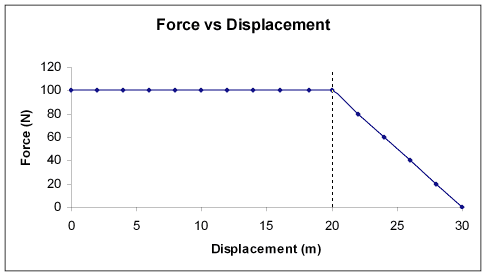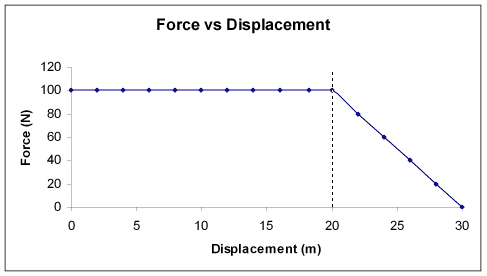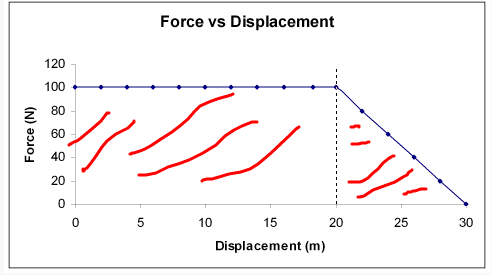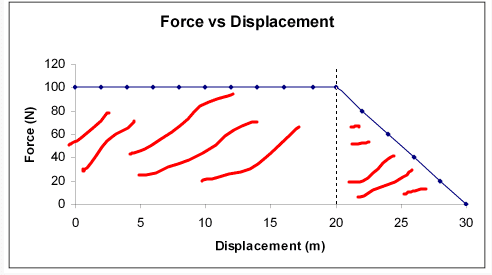# Force v Displacement graph

## Homework Statement

If the following Force v. Displacement graph is for a 9.9kg object and it's initial velocity is 28.6 m/s the what is its velocity at 30 m?## Homework Equations

I am not certain that i am even using the right equations with this but:

W = fd
KE = 1/2mv(final)^2 - 1/2mv(initial)^2

## The Attempt at a Solution

I thought since W=Fd and work is proportional to kinetic energy, which is
KE=1/2mv(final)^2 - 1/2mv(initial)^2 and i then could substitute in the Fd, making the new equation:

Fd = 1/2mv(final)^2 - 1/2mv(initial)^2

This could then be rearranged to find final velocity:

sqrt { (Fd + 1/2mv(initial)^2) / 1/2m } = v(final)

Only the issue is that i do not know which F to use to find the final velocity at d=30meters. Because if i use the 0 the whole Fd cancels out, and i get the wrong answer. But if i use 100 then it comes out as larger than the initial velocity, which is not possible as it is losing speed due to lack of force after 20 meters.

## Answers and Replies

If the following Force v. Displacement graph is for a 9.9kg object and it's initial velocity is 28.6 m/s the what is its velocity at 30 m?

Actually work done is a scalar product of force and displacement vector and the amount of work done is sored with the body as kinetic energy so calculate the work done from the graph and use it to find the final velocity.

•df102015
Actually work done is a scalar product of force and displacement vector and the amount of work done is sored with the body as kinetic energy so calculate the work done from the graph and use it to find the final velocity.

thanks, haha so i was way off, isn't work then supposed to be the area under the line on the graph?

## Homework Statement

If the following Force v. Displacement graph is for a 9.9kg object and it's initial velocity is 28.6 m/s the what is its velocity at 30 m?## Homework Equations

I am not certain that i am even using the right equations with this but:

W = fd
KE = 1/2mv(final)^2 - 1/2mv(initial)^2

## The Attempt at a Solution

I thought since W=Fd and work is proportional to kinetic energy, which is
KE=1/2mv(final)^2 - 1/2mv(initial)^2 and i then could substitute in the Fd, making the new equation:

Fd = 1/2mv(final)^2 - 1/2mv(initial)^2

This could then be rearranged to find final velocity:

sqrt { (Fd + 1/2mv(initial)^2) / 1/2m } = v(final)

Only the issue is that i do not know which F to use to find the final velocity at d=30meters. Because if i use the 0 the whole Fd cancels out, and i get the wrong answer. But if i use 100 then it comes out as larger than the initial velocity, which is not possible as it is losing speed due to lack of force after 20 meters.

As the force changes over time, The best way to find the work in graphs is by getting the areaYou have a triangle and a rectangle. Find the area and sum it up. You will end you will the the work done

•df102015
Only the issue is that i do not know which F to use to find the final velocity at d=30meters. Because if i use the 0 the whole Fd cancels out, and i get the wrong answer. But if i use 100 then it comes out as larger than the initial velocity, which is not possible as it is losing speed due to lack of force after 20 meters.

in the graph the value of force and displacement is given so calculate the work done

As the force changes over time, The best way to find the work in graphs is by getting the areaYou have a triangle and a rectangle. Find the area and sum it up. You will end you will the the work done

And do i use the numbers of the x and y axes to find area? in this case the rectangle being 100x20 and the triangle as 1/2x100x10 ?

i keep getting an answer larger than the initial velocity of 28.6, is that at all possible?

As the force changes over time, The best way to find the work in graphs is by getting the areaYou have a triangle and a rectangle. Find the area and sum it up. You will end you will the the work done

I got 2500 for the work, is that correct?

I havent calculated the velocity but it should be larger than the initial. Work is adding energy to the system. You add more energy this energy will be kinetic. While the mass and 0.5 obviously dont change then the only thing that will change is the velocity.

and the work is 2500 right :D

So yes it should be larger.

Chestermiller
Mentor
I got 2500 for the work, is that correct?
Yes. So, show us your detailed calculations for getting the final velocity. Incidentally, what are the units.

•df102015
Yes. So, show us your detailed calculations for getting the final velocity. Incidentally, what are the units.

W = 1/2mv(final)^2 - 1/2mv(initial)^2
2500 = (0.5 x 9.9 x V^2) - (0.5 x 9.9 x 28.6^2)
2500 = 4.95V^2 - 4048.9
6548.9 = 4.95V^2
1323.0 = V^2
36.4 = v(final)

Units:
W is in Joules
Mass is in kilograms
Velocity is in meters per second
Displacement is in meters
Force is in newtons

Chestermiller
Mentor
I didn't check your arithmetic, but your approach looks OK.

•drvrm and df102015
I didn't check your arithmetic, but your approach looks OK.

Thank you, i got the right answer, just checked it! (:

•drvrm